# The input source v,(t)The output voltage v,(t) = -10 sin(wt).10 cos (wt).HE0.1 F0.1 Hv(t)vo(t)1Ω

Question
21 views

1.By using phasor, find the value of w.
2.By using time domin (no phasor) , find the          value of w.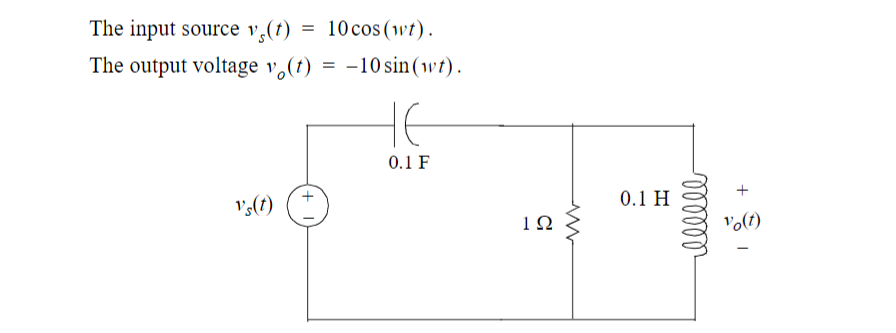help_outlineImage TranscriptioncloseThe input source v,(t) The output voltage v,(t) = -10 sin(wt). 10 cos (wt). HE 0.1 F 0.1 H v(t) vo(t) 1Ω fullscreen
check_circle

Step 1

(1)

Given values are –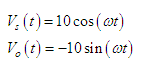Given circuit is –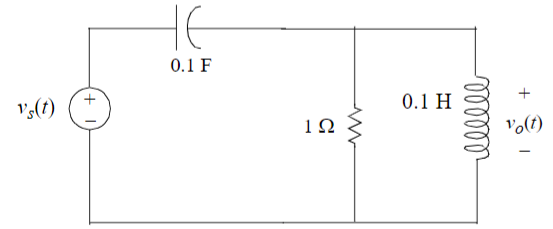Step 2

Output voltage is also written as –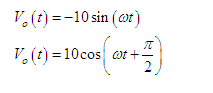Phasor diagram between input and output voltage is –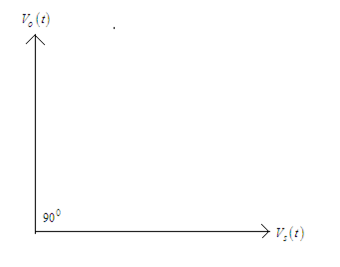Phase difference between input and output voltage is 90 degrees.

Step 3

Modified circuit is –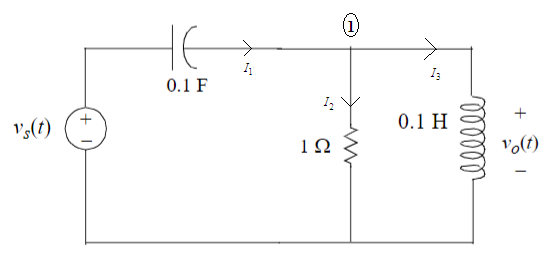Applying KCL at node (1) –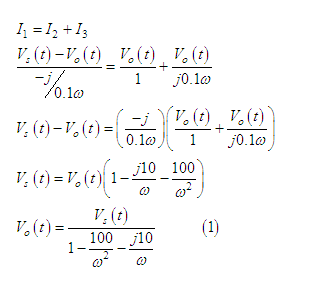...

### Want to see the full answer?

See Solution

#### Want to see this answer and more?

Solutions are written by subject experts who are available 24/7. Questions are typically answered within 1 hour.*

See Solution
*Response times may vary by subject and question.
Tagged in